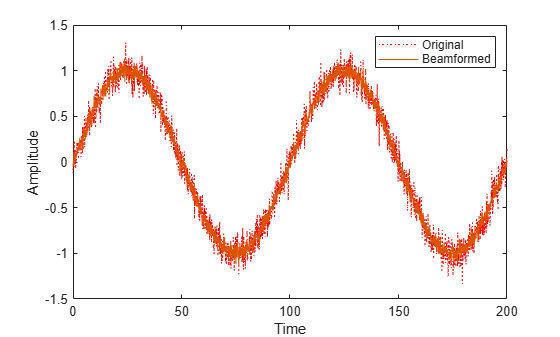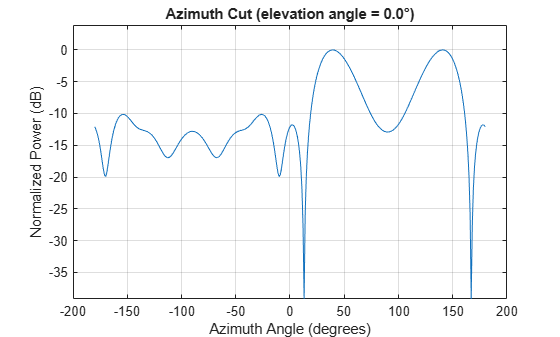# phased.MVDRBeamformer

Narrowband minimum-variance distortionless-response beamformer

## Description

The phased.MVDRBeamformer System object™ implements a narrowband minimum-variance distortionless-response (MVDR) beamformer. The MVDR beamformer is also called the Capon beamformer. An MVDR beamformer belongs to the family of constrained optimization beamformers.

To beamform signals arriving at an array:

1. Create the `phased.MVDRBeamformer` object and set its properties.

2. Call the object with arguments, as if it were a function.

## Creation

### Syntax

``beamformer = phased.MVDRBeamformer``
``beamformer = phased.MVDRBeamformer(Name,Value)``

### Description

````beamformer = phased.MVDRBeamformer` creates an MVDR beamformer System object, `beamformer`, with default property values.```
````beamformer = phased.MVDRBeamformer(Name,Value)` creates an MVDR beamformer with each property `Name` set to a specified `Value`. You can specify additional name-value pair arguments in any order as (`Name1`,`Value1`,...,`NameN`,`ValueN`). Enclose each property name in single quotes.Example: ```beamformer = phased.MVDRBeamformer('SensorArray',phased.URA,'OperatingFrequency',300e6)``` sets the sensor array to a uniform rectangular array (URA) with default URA property values. The beamformer has an operating frequency of 300 MHz.```

## Properties

expand all

Unless otherwise indicated, properties are nontunable, which means you cannot change their values after calling the object. Objects lock when you call them, and the `release` function unlocks them.

If a property is tunable, you can change its value at any time.

Sensor array, specified as an array System object belonging to Phased Array System Toolbox. The sensor array can contain subarrays.

Example: `phased.URA`

Signal propagation speed, specified as a real-valued positive scalar. Units are in meters per second. The default propagation speed is the value returned by `physconst('LightSpeed')`.

Example: `3e8`

Data Types: `single` | `double`

Operating frequency, specified as a positive scalar. Units are in Hz.

Example: `1e9`

Data Types: `single` | `double`

Diagonal loading factor, specified as a nonnegative scalar. Diagonal loading is a technique used to achieve robust beamforming performance, especially when the sample size is small. A small sample size can lead to an inaccurate estimate of the covariance matrix. Diagonal loading also provides robustness due to steering vector errors. The diagonal loading technique adds a positive scalar multiple of the identity matrix to the sample covariance matrix.

Tunable: Yes

Data Types: `single` | `double`

Enable training data input, specified as `false` or `true`. When you set this property to `true`, use the training data input argument, `XT`, when running the object. Set this property to `false` to use the input data, `X`, as the training data.

Data Types: `logical`

Source of beamforming direction, specified as `'Property'` or ```'Input port'```. Specify whether the beamforming direction comes from the `Direction` property of this object or from the input argument, `ANG`. Values of this property are:

 `'Property'` Specify the beamforming direction using the `Direction` property. `'Input port'` Specify the beamforming direction using the input argument, `ANG`.

Data Types: `char`

Beamforming directions, specified as a real-valued 2-by-1 vector or a real-valued 2-by-L matrix. For a matrix, each column specifies a different beamforming direction. Each column has the form `[AzimuthAngle;ElevationAngle]`. Azimuth angles must lie between –180° and 180° and elevation angles must lie between –90° and 90°. All angles are defined with respect to the local coordinate system of the array. Units are in degrees.

Example: `[40;30]`

#### Dependencies

To enable this property, set the `DirectionSource` property to `'Property'`.

Data Types: `single` | `double`

The number of bits used to quantize the phase shift component of beamformer or steering vector weights, specified as a nonnegative integer. A value of zero indicates that no quantization is performed.

Example: `5`

Data Types: `single` | `double`

Enable the output of beamforming weights, specified as `false` or `true`. To obtain the beamforming weights, set this property to `true` and use the corresponding output argument, `W`. If you do not want to obtain the weights, set this property to `false`.

Data Types: `logical`

## Usage

### Syntax

``Y = beamformer(X)``
``Y = beamformer(X,XT)``
``Y = beamformer(X,ANG)``
``Y = beamformer(X,XT,ANG)``
``[Y,W] = beamformer(___)``

### Description

example

````Y = beamformer(X)` performs MVDR beamforming on the input signal, `X`, and returns the beamformed output in `Y`. This syntax uses `X` as training samples to calculate the beamforming weights.```
````Y = beamformer(X,XT)` uses `XT` as training samples to calculate the beamforming weights. To use this syntax, set the TrainingInputPort property to `true`.```
````Y = beamformer(X,ANG)` uses `ANG` as the beamforming direction. To use this syntax, set the DirectionSource property to `'Input port'`.```
````Y = beamformer(X,XT,ANG)` combines all input arguments. To use this syntax, set the `TrainingInputPort` property to `true` and set the DirectionSource property to `'Input port'`.```
````[Y,W] = beamformer(___)` returns the beamforming weights, `W`. To use this syntax, set the WeightsOutputPort property to `true`.```

### Input Arguments

expand all

Input signal, specified as a complex-valued M-by-N matrix. N is the number of array elements. If the sensor array contains subarrays, N is the number of subarrays. If you set TrainingInputPort to `false`, M must be larger than N; otherwise, M can be any positive integer.

The size of the first dimension of the input matrix can vary to simulate a changing signal length. A size change can occur, for example, in the case of a pulse waveform with variable pulse repetition frequency.

Data Types: `single` | `double`
Complex Number Support: Yes

Training data, specified as a complex-valued P-by-N matrix. If the sensor array contains subarrays, N is the number of subarrays; otherwise, N is the number of elements. P must be larger than N.

The size of the first dimension of the input matrix can vary to simulate a changing signal length. A size change can occur, for example, in the case of a pulse waveform with variable pulse repetition frequency.

Example: `[1 0.5 2.6; 2 -0.2 0; 3 -2 -1]`

#### Dependencies

To enable this argument, set the TrainingInputPort property to `true`.

Data Types: `single` | `double`
Complex Number Support: Yes

Beamforming directions, specified as a real-valued 2-by-1 column vector, or 2-by-L matrix. L is the number of beamforming directions. Each column has the form `[AzimuthAngle;ElevationAngle]`. Units are in degrees. Each azimuth angle must lie between –180° and 180°, and each elevation angle must lie between –90° and 90°.

Example: `[40;10]`

#### Dependencies

To enable this argument, set the DirectionSource property to `'Input port'`.

Data Types: `single` | `double`

### Output Arguments

expand all

Beamformed output, returned as a complex-valued M-by-L matrix, where M is the number of rows of `X` and L is the number of beamforming directions.

Data Types: `single` | `double`
Complex Number Support: Yes

Beamforming weights, returned as a complex-valued N-by-L matrix. If the sensor array contains subarrays, N is the number of subarrays; otherwise, N is the number of elements. L is the number of beamforming directions.

#### Dependencies

To enable this output, set the WeightsOutputPort property to `true`.

Data Types: `single` | `double`
Complex Number Support: Yes

## Object Functions

To use an object function, specify the System object as the first input argument. For example, to release system resources of a System object named `obj`, use this syntax:

`release(obj)`

expand all

 `step` Run System object algorithm `release` Release resources and allow changes to System object property values and input characteristics `reset` Reset internal states of System object

## Examples

collapse all

Apply an MVDR beamformer to a 5-element ULA. The incident angle of the signal is 45 degrees in azimuth and 0 degree in elevation. The signal frequency is .01 hertz. The carrier frequency is 300 MHz.

```t = [0:.1:200]'; fr = .01; xm = sin(2*pi*fr*t); c = physconst('LightSpeed'); fc = 300e6; rng('default'); incidentAngle = [45;0]; array = phased.ULA('NumElements',5,'ElementSpacing',0.5); x = collectPlaneWave(array,xm,incidentAngle,fc,c); noise = 0.1*(randn(size(x)) + 1j*randn(size(x))); rx = x + noise;```

Compute the beamforming weights.

```beamformer = phased.MVDRBeamformer('SensorArray',array,... 'PropagationSpeed',c,'OperatingFrequency',fc,... 'Direction',incidentAngle,'WeightsOutputPort',true); [y,w] = beamformer(rx);```

Plot the signals.

```plot(t,real(rx(:,3)),'r:',t,real(y)) xlabel('Time') ylabel('Amplitude') legend('Original','Beamformed')```Plot the array response pattern using the MVDR weights.

```pattern(array,fc,[-180:180],0,'PropagationSpeed',c,... 'Weights',w,'CoordinateSystem','rectangular',... 'Type','powerdb');```## Algorithms

expand all

 Van Trees, H. Optimum Array Processing. New York: Wiley-Interscience, 2002.

 Frost, O. “An Algorithm For Linearly Constrained Adaptive Array Processing”, Proceedings of the IEEE. Vol. 60, Number 8, August, 1972, pp. 926–935.